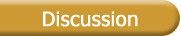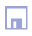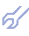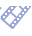Perceptrons and neural networks Perceptron with two layers of links, connecting S-A and A-R units, designed by Prof. Frank Rosenblatt, is basic pioneer network for pattern recognition. Similar multilayered algorithms for processes forecasting are known as GMDH parametric algorithms. Second F.Rosenblatt theorem is fundamental base for all pattern recognition neural networks. But his proposals for perceptrons links learning seems now not effective ones: there was proposed to vary number of hidden A-units and choose the coefficients of S-A links by random way. Now is clear that number of A-units should be equal to number of realizations in learning sub-sample. Then it is easy to choose links S-A coefficients to get zero error on all realizations of this sub-sample; instead of A-R links should be used Indicator of Minimal Value (IMV) . Information about which A-unite produce minimal signal can be used for accurate solution of pattern recognition problems. We can conclude that alpha- and beta-learning procedures, developed by F.Rosenblatt, as well back-propagation learning are not necessary for perceptrons design. Perceptrons can be simply calculated directly by algebraic way. We consider linear and some non-linear equations, which can be linearized for small deflections from equilibrium points. Generally all procedures of iteration type should be replaced by solution of a system of Gauss normal equations because there is no any constrains on number of realizations which we can take from data sample. Ordinary single-layered neural network can be considered as a committee of perceptrons. Here instead of one equation, a system of them should be solved. But all iteration type learning procedures can be excluded as less effective. Algebraic calculation solves all the questions of single-multilayered neural network design. Twice-multilayered neural networks with active neurons unite perceptrons (for pattern recognition) or GMDH algorithms (for forecasting and interpolation) into multilayered structure. Mathematical description of neural network with active neurons is a system of equation systems which provide accurate algebraic solution. To use any iterative procedures like stochastic approximation or back propagation simply are nonsense. Algebraic calculation gives zero error on all realizations of learning sub-sample. But when we want to minimize error calculated on future samples, which will be received in near future, an optimal clusterization of learning sub-sample should be fulfilled. Coordinates of clusters centers should be used as sample of filtrated from noise initial data. This is result of ideas of the optimal physical clusterization concept.